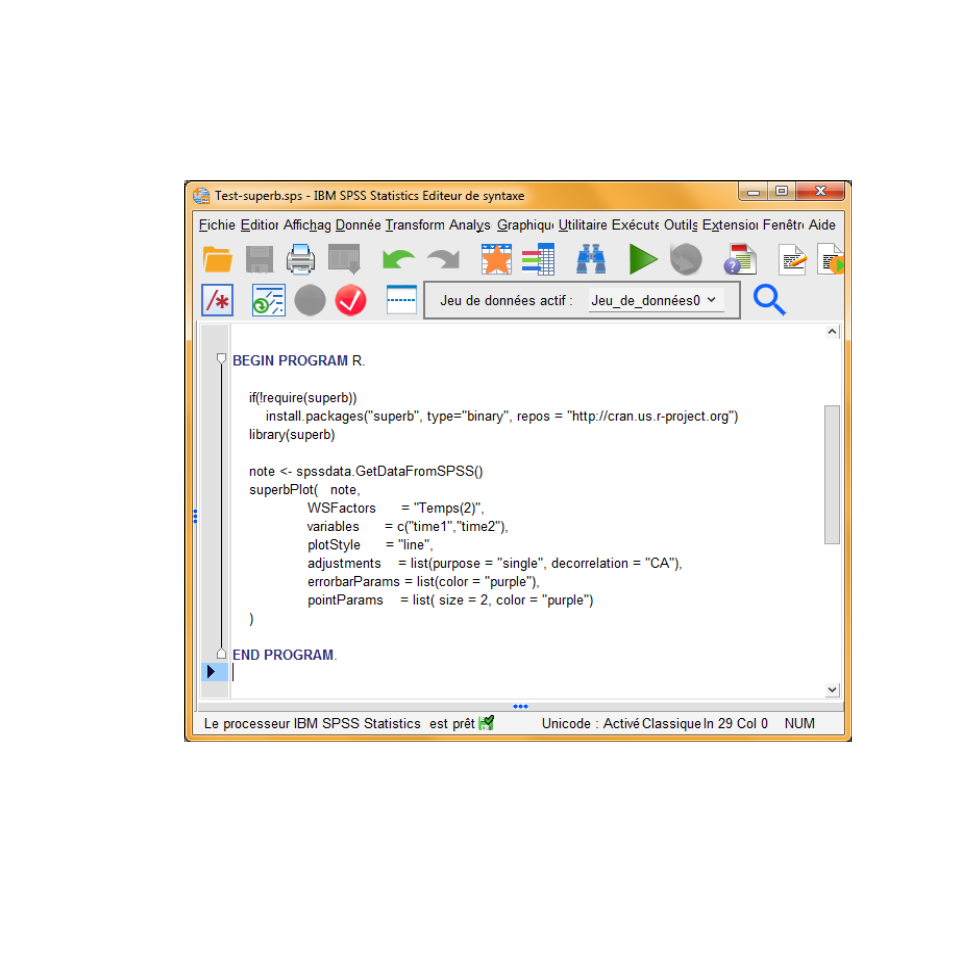The purpose of superb it to make plots showing adjusted error bars. However, not everyone are using R. Luckily, for those of you who are using SPSS, you have three options: 1) R can read SPSS file and therefore, you can open the data and make the plot in R before returning to SPSS. 2) It is possible to use SPSS only –from a syntax window– get access to all the capabilities of superb. 3) Using the graphical user interface superbShiny, you can also open SPSS files. This interface is available online as well.

In this vignette, we demonstrate how to use these three options to make a plot with SPSS data.

## First option: Using R

Suppose that you have a file called SPSS_Demo.sav. Using the library foreign, you can open the file and use it in R to make your plot.

The following syntax for example will open an SPSS file, assuming it is in a folder on your hard drive C:. First, set the working directory and the file name with

setwd("c:")
file <- "Demo_SPSS.sav"

Then you are ready to read the file with the help of the foreign library

library(foreign)
data <- read.spss(file, to.data.frame = TRUE)
## re-encoding from UTF-8

Finally, ask for a plot with

superbPlot( data,
WSFactors      = "Temps(2)",
variables      = c("time1","time2"),
plotStyle      = "line",
decorrelation = "CA"),
errorbarParams = list(color = "purple"),
pointParams    = list( size = 2, color = "purple")
)

## Second option: Using SPSS

The recent versions of SPSS comes bundled with an R interpretor. Further, if you open a Syntax window (menu File: New: Syntax), you can send R instructions enclosed within BEGIN PROGRAM R. and END PROGRAM.

To know if your SPSS installation has R installed, you could for example type these instructions:

BEGIN PROGRAM R.
# a quick test
cat("R is up and running: ", R.version.string, "\n")
END PROGRAM.

then select them all and press Ctrl-R to execute. It R is accepted within SPSS, you should see an ouptut indicating the version of R installed. It has to be R above 4.0.

If things are working, then you are ready to make your plot with

BEGIN PROGRAM R.
# this will install superb if needed; may take a few minutes
if(!require(superb))
install.packages("superb", type="binary", repos = "http://cran.us.r-project.org")

# set the library to be in used
library(superb)

# transfer the data from SPSS into R
data <- spssdata.GetDataFromSPSS()

# all good! make a plot using superbPlot()
superbPlot(   data,
WSFactors      = "Temps(2)",
variables      = c("time1","time2"),
plotStyle      = "line",
adjustments    = list(purpose = "single", decorrelation = "CA"),
errorbarParams = list(color = "purple"),
pointParams    = list( size = 2, color = "purple")
)

END PROGRAM.

Here are screen captures showing the syntax window:Figure 2: Syntax to generate a plot

## Third option: Using a graphical user interface

A graphical user interface is available at this link

This interface can read a few file format, including SPSS files. You can consult a Youtube demonstration here.

## In summary

The superb framework can be used to display any summary statistics. Here, we showed how superbPlot() can be used with SPSS datasets.

I thank Michael Cantinotti for raising my awarness to the fact that new versions of SPSS can show plots produced within BEGIN PROGRAM R. and END PROGRAM. syntax lines and providing a short example.